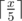Practice

CakeWalk

# PREREQUISITES:

None at all# PROBLEM:

what is the minimum number of steps Bean needs to make in order to get to his friend’s house? In one step the Bean can move 1,2,3,4, or 5 positions forward.

# EXPLANATION:

You have to make given Integer Z equal to zero in the minimum number of operations

You can do it by subtracting the maximum number out of 1,2,3,4 or 5 from Z until Z becomes strictly less than that number or if Z becomes zero print the count else repeat the same with other remaining numbers until Z becomes 0.

ALTERNATE WAY
It’s optimal to do the biggest possible step every time. So Bean should do several steps by distance 5 and one or zero step by smaller distance. Answer equals to# SOLUTIONS:

Solution 1
``````#include <bits/stdc++.h>
using namespace std;
int main()
{
int t;
cin >> t;
while (t--)
{
int x;
cin >> x;

int count = 0;
while (x != 0)
{
if (x >= 5)
{
x -= 5;
}
else if (x >= 4)
{
x -= 4;
}
else if (x >= 3)
{
x -= 3;
}
else if (x >= 2)
{
x -= 2;
}
else if (x >= 1)
{
x -= 1;
}
count++;
}
cout << count << endl;
}
return 0;
}
``````
Solution 2
``````#include <iostream>
using namespace std;
int main() {
int t;
cin>>t;
while(t--)
{
int x;
cin >> x;
cout << (x + 4) / 5 << '\n';
}
return 0;
}
``````